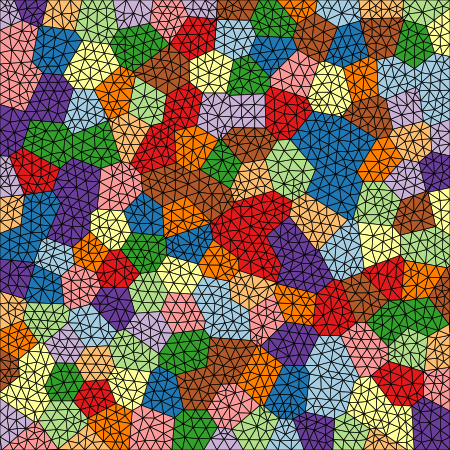# Minimal Example¶

## XML Input File¶

The basename for this file is `minimal_paired.xml`. The file can be run using this command:

```microstructpy --demo=minimal_paired.xml
```

The full text of the file is:

```<?xml version="1.0" encoding="UTF-8"?>
<input>
<material>
<shape> circle </shape>
<size> 0.09 </size>
</material>

<domain>
<shape> square </shape>
</domain>

<settings>
<directory> minimal </directory>
<plot_axes> False </plot_axes>
<color_by> seed number </color_by>
<colormap> Paired </colormap>
<mesher> gmsh </mesher>
<mesh_size> 0.03 </mesh_size>
</settings>
</input>
```

## Material¶

There is only one material, with a constant size of 0.09.

## Domain Geometry¶

The material fills a square domain. The default side length is 1, meaning the domain is greater than 10x larger than the grains.

## Settings¶

The function will output plots of the microstructure process and those plots are saved as PNGs. They are saved in a folder named `minimal`, in the current directory (i.e `./minimal`).

The axes are turned off in these plots, creating PNG files with minimal whitespace.

This example also demonstrates how to use gmsh to generate a mesh, using the `<mesher>` and `<mesh_size>` tags in the input file.

Finally, the seeds and grains are colored by their seed number, not by material.

## Output Files¶

The three plots that this file generates are the seeding, the polygon mesh, and the triangular mesh. These three plots are shown in Fig. 7 - Fig. 9.Fig. 7 Minimal example - seed geometries.¶Fig. 8 Minimal example - polygonal mesh.¶Fig. 9 Minimal example - triangular mesh.¶